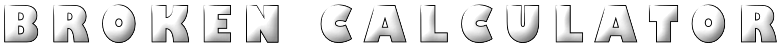###### 1 and 52 and 33 and 44 and 55 and 2More Calculator Activities

Use the keys on this broken calculator to make the totals from 1 to 20. Five has already been done as an example, see below.

 1 = 2 = 3 = 4 = 5 = 6 = 7 = 8 = 9 = 10 = 11 = 12 = 13 = 14 = 15 = 16 = 17 = 18 = 19 = 20 =

#### Currency ConversionTest your ability to convert from one currency to another with this self marking quiz. So far this activity has been accessed 5693 times and 464 people have earned a Transum Trophy for completing it.

## Broken Calculator 4

#### A challenge to produce the numbers from one to twenty on a calculator with some missing keys.

Click the keys on the calculator above to make the numbers 1 - 20. As you make each total your calculation will appear in the table on the right.

Make all twenty totals the earn a Transum Trophy.

Unlike a normal calculator, for this activity, you cannot use one total you have found to help make another. Each calculation starts from scratch (zero).

Try to make each total using the smallest number of calculator key presses.

Other broken calculators;
1 and 5 2 and 3 3 and 4 4 and 5 5 and 2 6 keys

Emma, Roundwood Primary School

Monday, November 19, 2012

"This is a good starter it really gets your brain moving but you could have the answers at the end of the page so you can check your answers. other then that it is a great puzzle on a fab website."

Transum,

Thursday, April 3, 2014

"Hi Emma, thanks for your comments about the Transum website. Very much appreciated. The solutions are available below for those signed into our teachers only access area. Suffice to say all of the totals are possible and it may be fun for the pupils to puzzle over the answers that they can't find rather than be tempted to peek at the solutions."

Grace, Stanwix

Wednesday, June 25, 2014

"Good starter what are the answers?

[Transum: The answers to most Transum activities are only available to teachers and parents who have signed in to their Transum account. You can apply for a subscription here."

Jane, Cambridge

Monday, September 15, 2014

"This is a fab starter but there seems to be a glitch. We typed in 25 - 5 -5 - 2 -2 -2 -2 x2 to make 14 and it kept coming up with the wrong answer of 5 - we tried it several times to make sure we weren't accidentally pressing any wrong digits and checked it on a normal calculator in the classroom to check we were right. It was annoying because 14 was the only number we had left to achieve.

[Transum: Thank you Jane for leaving a comment on this page. I would guess that the 'normal calculator' you have in the classroom does not follow the order of operations sometimes referred to with the acronym BIDMAS. Most calculators would do the multiplication in your calculation before they do all of the subtractions from left to right. The correct answer to the calculation you typed in is in fact 5.]"

Jojo, Gomez

Wednesday, February 24, 2016

"This is good. It is a great starter. But maybe you could add some more levels.I have completed everything already.
Thanks."

Friday, August 26, 2016

Josh, Inaburra

Sunday, March 4, 2018

"Hey,
Jane from Cambridge. The reason you can't do it is because the calculater uses order of operations so you would have to do multiplication first."